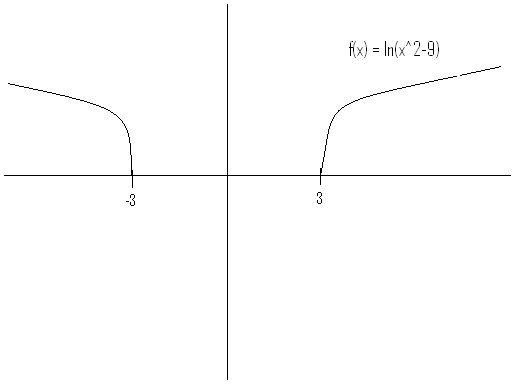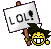3 Practice Free Response Problems...3 Practice Free Response Problems...

When you are posting a solution to any one of these problems, please give the year and problem number.

1975 AB 1:
Given the function f defined by f(x) = ln(x2 - 9).
(a) Describe the symmetry of the graph of f.

(b) Find the domain of f.

(c) Find all values of x such that f(x) = 0.

(d) Write a formula for f-1(x), the inverse function of f, for x > 3.

1975 AB 2:
A particle moves along the x-axis in such a way that its position at time t for t≥0 is given by
x(t) = 1/3t3 - 3t2 + 8t.
(a) Show that at time t = 0, the particle is moving to the right.

(b) Find all values of t for which the particle is moving to the left.

(c) What is the position of the particle at t = 3?

(d) When t = 3, what is the total distance the particle has traveled?

1975 AB 5:
The line x = c where c > 0 intersects the cubic y = 2x3 +3x2 - 9 at point P and the parabola y = 4x2 + 4x + 5 at point Q.
(a) If a line tangent to the cubic at point P is parallel to the line tangent to the parabola at point Q, find the value of c where c > 0.

(b) Write the equations of the two tangent lines described in (a).Supreme Math GOD

Posts : 22
Join date : 2009-03-19
Age : 37Re: 3 Practice Free Response Problems...

1975 AB 1:

a) The function f(x) = ln(x2-9) is reflected across the y-axis.b) The domain of ƒ is x < -3 or x > 3.
ln(x) exists when x > 0. So x2 -9 = 0 ⇒ x = ± 3

c)0 = ln(x2-9)
e0 = eln(x² -9)
1 = x2-9
x2 = 10
x = ±√(10)

d)x = ln(y2-9)
ex = eln(y^2-9)
ex = (y2-9)
ex+9 = y2
√(ex+9) = y = f -1

(Ok the corrected stuff is in orange. Wow....I can't believe I did that for part d xP I shouldn't do math late at night.)

1975 AB 2:

a) d/dt (1/3t3 - 3t2 + 8t) = t2 - 6t +8 = v(t)
(0)2 - 6(0) +8 = 8;
∴ v(t) = 8 > 0
If v(t) > 0, then the particle moves to the right.

b) If v(t) < 0, then the particle moves to the left.
t2 - 6t +8 < 0
(t - 4)(t - 2) < 0
2 < t < 4
∴ the particle is moving to the left when 2 < t < 4.

c) x(t) = 1/3t3 - 3t2 + 8t
1/3(3)3 - 3(3)2 + 8(3) = 6
If t = 3, then x = 6.

d)When t = 3, then the particle has traveled 6 units. (....Is this a trick question or something?)

Last edited by ebilmonky on Sun Jun 13, 2010 4:59 am; edited 2 times in total

ebilmonky

Posts : 8
Join date : 2010-06-02Re: 3 Practice Free Response Problems...

(d) Look at your second to third lines...what's wrong?

(c) Correct!Supreme Math GOD

Posts : 22
Join date : 2009-03-19
Age : 37Re: 3 Practice Free Response Problems...

1975 AB1

d)
x=ln(y2-9)
ex=y2-9
ex+9=y2
√(ex+9)=y=f-1(x)jodo_059

Posts : 11
Join date : 2010-06-02Re: 3 Practice Free Response Problems...

Well done! Now, can someone justify parts (a) and (b)?Supreme Math GOD

Posts : 22
Join date : 2009-03-19
Age : 37Re: 3 Practice Free Response Problems...

1975 AB1
a.) f(x)=ln(x^2-9) let x=-x
f(-x)=ln(-x^2-9)
f(-x)=ln(x^2-9) =f(x)=ln(x^2-9)
because f(-x)=f(x) it is an even graph so it reflects about the y axis

b.) x^2-9=0
x^2=3
x=±√9
x=± 3
so x≥3 and x≤-3

c.) 0=ln(x^2-9)
e^0=e^ln(x^2-9) --> e^0=1
1=x^2-9
10=x^2
x=±√10

d.) f(x)=ln(x^2-9); f(x)=y ; switch x and y
x=ln(y^2-9)
e^x=e^ln(y^2-9)
e^x=y^2-9
e^x+9=y^2
y=±√e^x+9=f-1(x)
f -1(x)=±√e^x+9

chiko

Posts : 2
Join date : 2010-06-04Re: 3 Practice Free Response Problems...

1975 AB 5:

(a) Tangents parallel when derivatives are equal
d/dx 2x^3 + 3x^2 - 9 = d/dx 4x^2 + 4x + 5
6x^2 + 6x = 8x + 4
6x^2 - 2x - 4 = 0
3x^2 - x - 2 = 0
(3x + 2)(x - 1) = 0
x = 1 or x = -2/3
x = c; c > 0 so c = 1

(b) 6x^2 + 6x = 8x + 4
6(1)^2 + 6(1) = 8(1) + 4
12 = 12
Slope = 12

y = 2x^3 + 3x^2 - 9____________y = 4x^2 + 4x + 5
y = 2(1)^3 + 3(1)^2 - 9_________y = 4(1)^2 + 4(1) + 5
y = -4________________________y = 13

y + 4 = 12(x - 1)_______________y - 13 = 12(x - 1)

Hopefully it's right ... how do make exponents and spaces?G-Unit

Posts : 3
Join date : 2010-06-11Re: 3 Practice Free Response Problems...

Fantastic JOB!!! Score: 9/9.Supreme Math GOD

Posts : 22
Join date : 2009-03-19
Age : 37NEW PROBLEMS...

AGAIN...NO CALCULATORS!!! Note that 1975 AB 2 is still open:

1975 AB 6/BC 2:

Let R be the region in the first quadrant bounded by the graphs of x2/9 + y2/81 = 1 and 3x + y = 9.

(a) Set up but do not evaluate an integral representing the area of R. Express the integrand a s a function of a single variable.

(b) Set up but do not evaluate an integral representing the volume of the solid generated when R is rotated about the x - axis. Express the integrand as a function of a single variable.

(c) Set up but do not evaluate an integral representing the volume of the solid generated when R is rotated about the y - axis. Express the integrand as a function of a single variable.

1975 BC 7 :

(a) For what value of m is the line y = mx tangent to the graph of y = lnx.

(b) Prove that the graph of y = lnx lies entirely below the graph of the line found in part (a).

(c) Use the results of (b) to show that ex≥xe for x > 0.

1976 AB 3/BC 2:
Let R be the region bounded by the curves f(x) = 4/x and g(x) = (x - 3)2.

(a) Find the area of R.

(b) Find the volume of the solid generated by revolving R about the x - axis.

*CHALLENGE PROBLEM #1 This is REALLY HARD

A snowplow can remove snow at a constant rate (in ft3/min). One day, there was no snow on the ground at sunrise, but sometime in the morning it began snowing at a steady rate. At noon, the plow began to remove snow. It had cleared 2 miles of snow between noon and 1 PM, and 1 more mile of snow between 1 PM and 2 PM. At what time did it start snowing?

HINTS:
(1) The velocity that the snowplow travels is inversely proportional to the amount of time since it started snowing.

(2) Note that ds/dt = k/t for some constant k (where s is the distance traveled by the plow).

*All Challenge Problems are about 100,000 times harder than the actual AP TEST.Supreme Math GOD

Posts : 22
Join date : 2009-03-19
Age : 371975 AB 2

a) x(t) =1/3t3 - 3t2 +8t
v(t) = t2 - 6t +8
v(0) = 8.
8>0, therefore particle is moving to the right.shakalohana

Posts : 17
Join date : 2010-06-041975 AB 6/BC 2

1975 AB 6/BC 2
The two equations are of a vertical ellipse and a line which create a sort of diagonal half oval in the first quadrant with its tips on points (0,9) and (3,0)

a) I used a veritcal rectangular strip so its Δx so therefore everything must be changed into only x so solve for y and do top(ellispse) minus bottom(line).
Therefore solve for y for both equations and you get:
y=√(81-9x2)
y=-3x+9

Then you plug it in and get:
∫ √(81-9x2)-(-3x+9) dx where a=0, b=3

b) Again i used a vertical rectangular strip and got a washer where R is the ellipse and r is the line. Plugging it in you get:
∫ π(√(81-9x2))2-(-3x+9)2 dx where a=0, b=3

c)Again i used a vertical rectangular strip and got a cylinder where r=x and h=ellispse-line. Plugging it in you get:
∫ 2πx[√(81-9x2)-(-3x+9)]dx where a=0 and b=3

BTW THOSE THINGS THAT LOOK LIKE n'S R PI.seikochan

Posts : 1
Join date : 2010-06-02Re: 3 Practice Free Response Problems...

1975 AB 2
(a) Correct - 2/2
Finish the rest now...

1975 AB 6/BC 2
Absolutely CORRECT! GREAT JOB! Score - 9/9Supreme Math GOD

Posts : 22
Join date : 2009-03-19
Age : 37Re: 3 Practice Free Response Problems...

the rest!
b)
v(t) = t2 - 6t + 8 = 0
(t-2)(t-4) = 0
t = 2, 4
so t moves to the left when greater than or equal to 2 and less than 4 because when it was at 0 it was moving to the right and so at 2 and 4 is when the particle stops and turns around.

c) so the position function is x(t) = 1/3t3 - 3t2 + 8t
and plugging in 3 for t, i got 15.

d) from when t=0 to t=3, i know that the particle only turns around once, which is at t=2.
so i have to do |x(2) - x(0)| + |x(3)- x(2)|. and i got 25/3 + 20/3 = 55/3shakalohana

Posts : 17
Join date : 2010-06-04Re: 3 Practice Free Response Problems...

Part (b) only asks for the time that the particle turns to the left, so v(t) < 0 ⇒ 2 < t < 4.

Part (c) OMG...TRY AGAIN!

Part (d) Correct!

Score: 8/9 Fix part (c)Supreme Math GOD

Posts : 22
Join date : 2009-03-19
Age : 37Re: 3 Practice Free Response Problems...

lolol did i calculate wrong!?! o.o

haha ok let me do this slowly...
1/3(27) - 3(9) + 8(3) = 9 - 27 + 24 = 6
hahahaha ok it's SIX.

BETTER!shakalohana

Posts : 17
Join date : 2010-06-04Re: 3 Practice Free Response Problems...